> > Tangential or curvature continuous condition

Tangential or curvature continuous condition
Create a smooth transition between curves or between surfaces by using a connect condition: tangential or curvature continuous. With 3D curves, the tangent or curvature value defined at the point on the edge or face determines the tangent or curvature of the curve to be created or modified. With Surfacing, the curvature value defined on the surface determines the curvature of the surface to be created.
Size of curve
Flat
Big
Turns strongly
Small
Straight line
Circle
In the image below, the top curve is flat with a big radius. The bottom curve turns and has a small radius.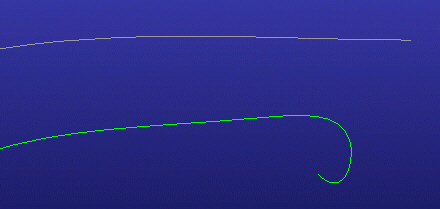When you specify a tangential or curvature continuous condition at a specific point,
The new curve will be tangential or curvature continuous at that point.
The tangent or the tangent and curvature will be smooth.
If only a tangential condition is specified, the curvature will not be smooth.
A tangential condition will maintain the same tangent direction.
A curvature continuous condition will maintain the same tangent direction and the same curvature radius.
To visualize the curvature (radius), use the Curve Analysis function.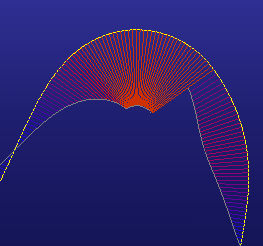In this example of Curve Analysis, the top curve is a circle and either side is a curve connected to the circle.
The curve on the left was connected with a curvature continuous condition. The smooth connection is shown by the way the red lines continue uninterrupted from one curve to the next. The curve on the right was connected with a tangential condition and shows a less smooth connection. The analysis conveys a jump in the curve by the way the red lines from the side curve do not match the red lines from the top curve.
Surfacing
To visualize the transition between two surfaces, use the Surface Analysis function.
 Tangential transitionThe stripes match, but are not smooth between surfaces.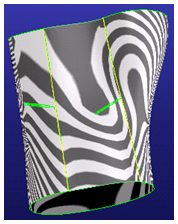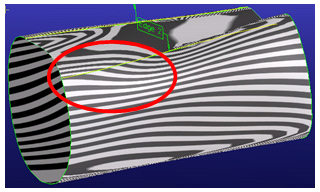Curvature continuous transitionThe stripes match and are smooth between the two surfaces.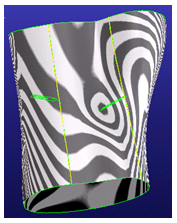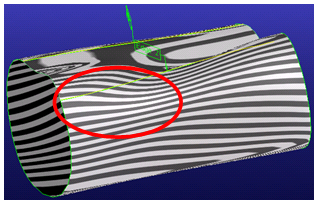In this example of Surface Analysis, the Bridge command created the surface between the two yellow lines. The Zebra Stripes analysis shows the difference between the new surface and adjacent surfaces with Tangential and Curvature continuity. The curvature continuous transition is more smooth than the tangential transition.
Limitations
In some cases,
It is not possible geometrically to create a curvature continuous transition.
A perfect curvature continuous transition would result in an expensive surface (the amount of data is large).
The results of a curvature continuous transition depend on the quality of the adjacent surfaces to which the new surfaces are added.
Curvature continuous transitions are approximately curvature continuous. Verify the transition with the Surface Analysis tool.